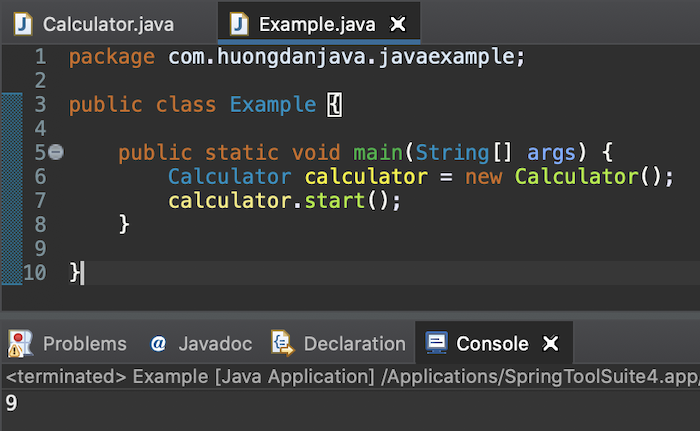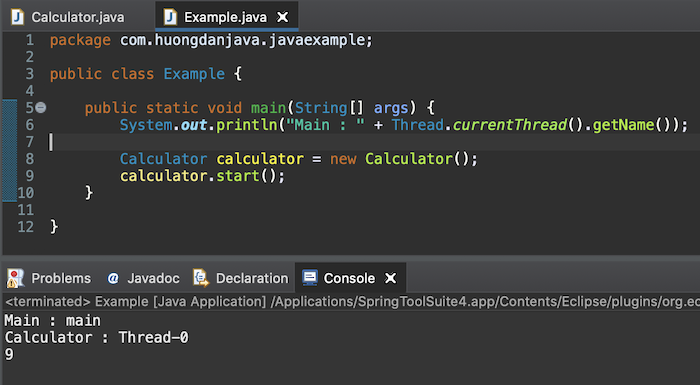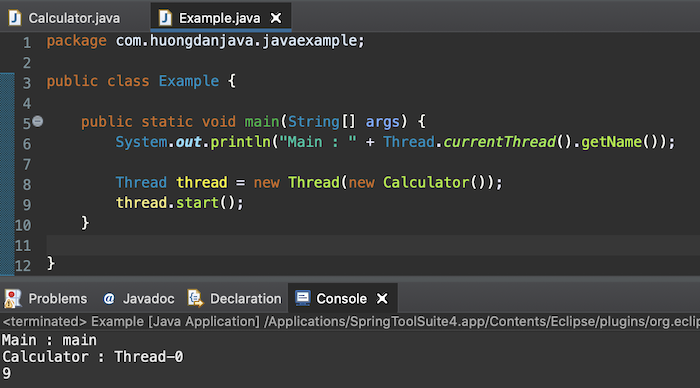# Initializing and running a thread in Java

In Java, we have two ways to initialize and run a thread, that is using the Thread class or the Runnable interface. How is it in detail? In this tutorial, we will learn about them together!

You can easily create a new thread by extending your class from the Thread class and then overriding the run() method of this Thread class. The code inside the run() method will be the task that we need to execute in the new thread.

For example, if I want to create a new thread to calculate the sum of 2 numbers by extending the Thread class, I will create a new class that extends the Thread class and override the run() method as follows:

To run the thread in this case, we will create a new instance of the Calculator class and call the start() method of this object to run it. Eg:

Result:You can clearly see whether our code is executed in the main thread or another thread by adding some lines of code as follows:

Result:If you notice, there will be a question that if we call the run() method instead of the start() method of the Calculator class, what is the difference? The answer is that if you call the run() method, the code inside it will be executed in the same thread of the program instead of a new thread.

For example, if I now change to call the run() method in the main function of the application:

You will see the following result:### Runnable interface

With the Runnable interface, your class needs to implement this interface and implement the run() method defined in it.

In the above example, instead of extending from the Thread class, we can implement the Runnable interface as follows:

To create a new thread and run our application in this case, you need to create a new Thread class with the parameter of the class implementing our Runnable interface. Example is as follows:

The result is the same:### Summary

Whether using extend class Thread or implement interface Runnable depends on your needs, but I prefer implementing interface Runnable because in Java, we can implement many different interfaces but can only extend from a certain class.